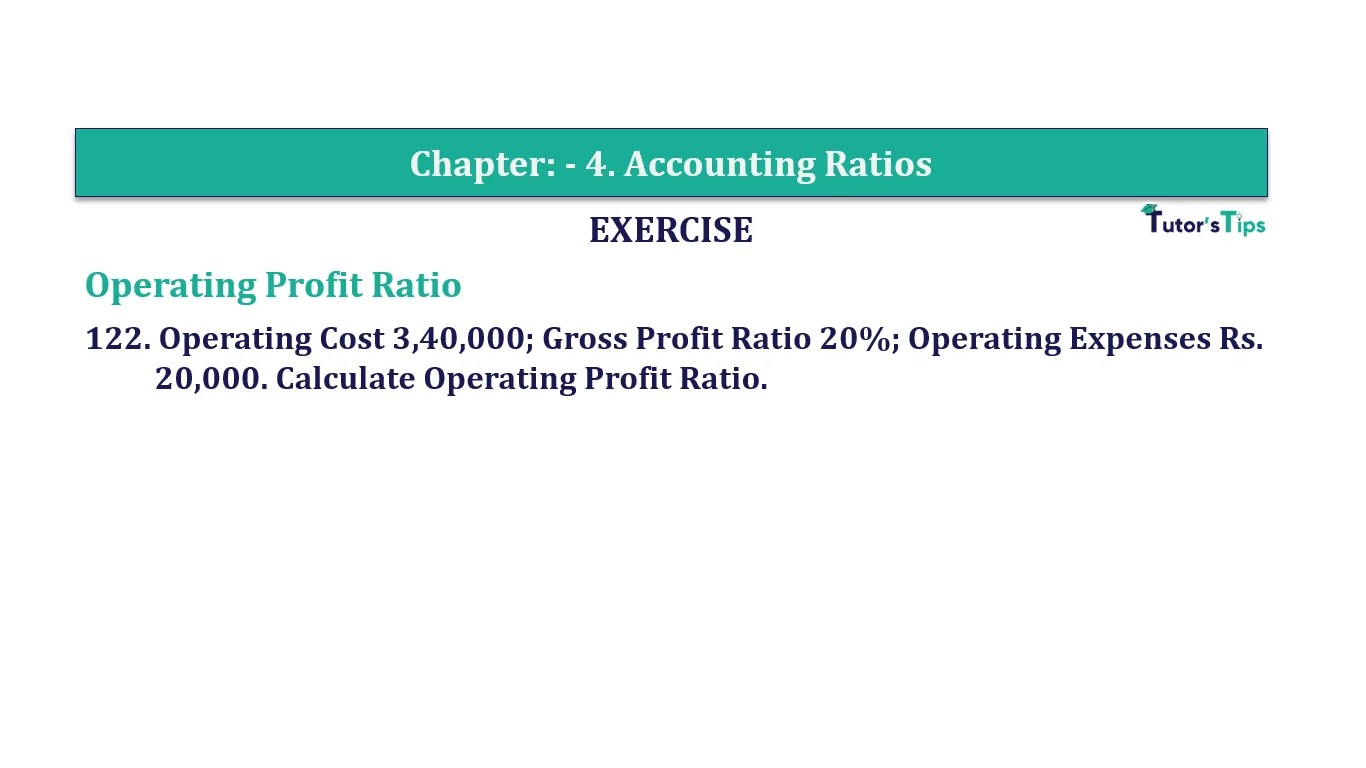# Question 122 Chapter 4 of +2-B – T.S. Grewal 12 ClassQuestion No. 122- Chapter No.4 - T.S. Grewal +2 Book Part B

Question 122 Chapter 4 of +2-B

Operating Ratio

122. Operating Cost 3,40,000; Gross Profit Ratio 20%; Operating Expenses Rs.
20,000. Calculate Operating Profit Ratio.

### The solution of Question 122 Chapter 4 of +2-B: –

 Cost of Revenue from Operations = Operating Cost-Operating Expenses = Rs. 3,40,000 – Rs. 20,000 = Rs. 3,20,000
 Gross Profit = 20 X Rs3,20,000 80 = Rs 80,000

 Revenue from Operations = Cost of Revenue from Operations + Gross Profit = Rs. 3,20,000 – Rs. 80,000 = Rs. 4,00,000 Operating Profit = Revenue from Operations – Operating Cost = Rs. 4,00,000 – Rs. 3,40,000 = Rs. 60,000

 Operating Ratio = Operating Cost X 100 Net Sales
 Operating Ratio = Rs. 60,0000 X 100 Rs. 4,00,000 = 15%

Balance Sheet: Meaning, Format & Examples

Comment if you have any question.

Also, Check out the solved question of previous Chapters: –